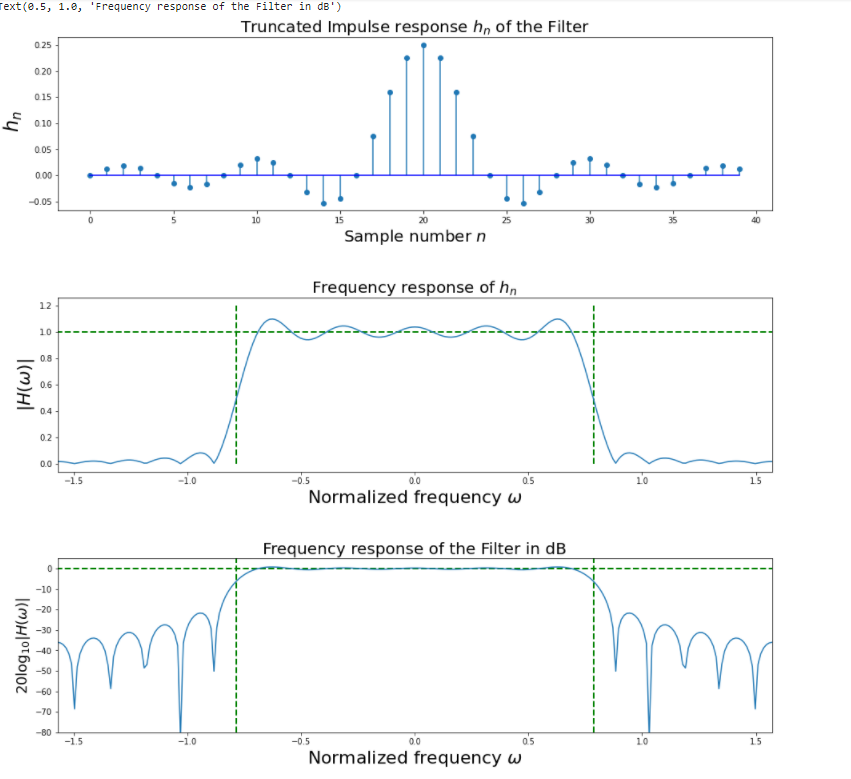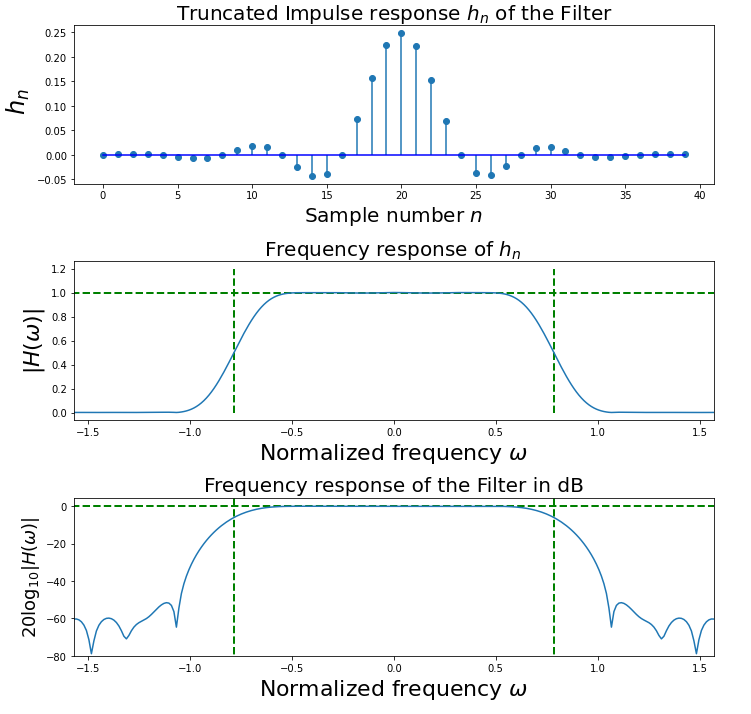# Gibb’s Phenomenon Rectangular and Hamming Window Implementation

• Last Updated : 23 Jun, 2021

What is Gibb’s Phenomenon?

Gibb’s Phenomenon in Digital Filter’s refer to the manner how a Fourier series of periodic functions behave near jump discontinuation, the partial sum of the Fourier series has large oscillations near the discontinuation, which might increase the maximum of the partial sum above that of the function itself. Thus, there is an overshoot as the terms are getting added of the Fourier series at the discontinuity.

In the signal processing terms, we can translate Gibb’s Phenomenon as the step response of the filter & the truncation of our signal via Fourier series can be termed as filtering out the signal.

We will be understanding this via example problem:

Specifications of the Filter

1. Digital Lowpass FIR filter with 41 taps.
2. Cutoff frequency of the filter is pi/4

We will be implementing the solution using both the Rectangular & Hamming Window Technique.

What is Rectangular Window Technique?

In the Rectangular window, the Fourier Transform converges to sinc function, giving a rectangular shape at the edges thus the name Rectangular Window. The Rectangular is not widely used in the industry for filtering signals.

What is the Hamming Window Technique?

Hamming Window is a far more optimized approach to filter signals as it cuts off signal points on either side for us to see the more clear picture of the signal’s frequency spectrum.

Step-by-step Approach:

Step 1: Importing all the necessary libraries.

## Python3

 ##import required libraryimport numpy as npimport scipy.signal as signalimport matplotlib.pyplot as plt

Step 2: Define variables with the given specifications of the filter.

## Python3

 #Given specificationwc =np.pi/4        #Cutoff frequency in radianN1=int(input())    #Given filter lengthM=(N1-1)/2         #Half length of the filter

Step 3: Computations to calculate the magnitude, phase response to get Rectangular Window coefficients

## Python3

 N = 512    ## Choose DFT sizen = np.arange(-M,M)  #Desired impulse response coefficients of the lowpass#filter with cutoff frequency wchd = wc / np.pi * np.sinc(wc * (n) / np.pi) #Select the rectangular window coefficientswin =signal.boxcar(len(n)) #Perform multiplication of desired coefficients and#window coefficients in the time domain#instead of convolving in the frequency domainh = hd * win # Modified filter coefficients ##Compute the frequency response of the modified filter coefficientsw,Hh = signal.freqz(h, 1, whole = True, worN = N)## get entire frequency domain##Shift the FFT to center for plottingwx = np.fft.fftfreq(len(w))

Step 4: Plotting the Truncated Impulse Response, Frequency Response, Frequency Response of the Filter  using The Rectangular Window Method

## Python3

 ##Plotting of the resultsfig,axs = plt.subplots(3, 1)fig.set_size_inches((16, 16))plt.subplots_adjust(hspace = 0.5) #Plot the modified filter coefficientsax = axsax.stem(n + M, h, basefmt =  'b-', use_line_collection = 'True')ax.set_xlabel("Sample number $n$", fontsize = 20)ax.set_ylabel(" $h_n$", fontsize = 24)ax.set_title('Truncated Impulse response $h_n$ of the Filter', fontsize = 20) #Plot the frequency response of the filter in linear unitsax = axsax.plot(w-np.pi, abs(np.fft.fftshift(Hh)))ax.axis(xmax = np.pi/2, xmin = -np.pi/2)ax.vlines([-wc,wc], 0, 1.2, color = 'g', lw = 2., linestyle = '--',)ax.hlines(1, -np.pi, np.pi, color = 'g', lw = 2., linestyle = '--',)ax.set_xlabel(r"Normalized frequency $\omega$",fontsize = 22)ax.set_ylabel(r"$|H(\omega)|$",fontsize = 22)ax.set_title('Frequency response of $h_n$ ', fontsize = 20) #Plot the frequency response of the filter in dBax=axsax.plot(w-np.pi, 20*np.log10(abs(np.fft.fftshift(Hh))))ax.axis(ymin = -80,xmax = np.pi/2,xmin = -np.pi/2)ax.vlines([-wc,wc], 10, -80, color = 'g', lw = 2., linestyle = '--',)ax.hlines(0, -np.pi, np.pi, color = 'g', lw = 2., linestyle = '--',)ax.set_xlabel(r"Normalized frequency $\omega$",fontsize = 22)ax.set_ylabel(r"$20\log_{10}|H(\omega)|$",fontsize = 18)ax.set_title('Frequency response of the Filter in dB', fontsize = 20)Step 5: Computations to calculate the magnitude, phase response to get Hamming Window coefficients

## Python3

 #Desired impulse response coefficients of the lowpass filter with cutoff frequency wchd=wc/np.pi*np.sinc(wc*(n)/np.pi)### START CODE HERE ### (≈ 1 line of code) #Select the rectangular window coefficientswin =signal.hamming(len(n))### START CODE HERE ### (≈ 1 line of code) #Perform multiplication of desired coefficients and window#coefficients in time domain#instead of convolving in frequency domainh = hd*win### START CODE HERE ### (≈ 1 line of code ## Modified filter coefficients ##Compute the frequency response of the modified filter coefficientsw,Hh =signal.freqz(h,1,whole=True,worN=N)### START CODE HERE ### (≈ 1 line of code)     ## get entire frequency domain ##Shift the FFT to center for plottingwx =np.fft.fftfreq(len(w))### START CODE HERE ### (≈ 1 line of code)

Step 6: Plotting the Truncated Impulse Response, Frequency Response, Frequency Response of the Filter  using The Hamming Window Method

## Python3

 ##Plotting of the resultsfig,axs = plt.subplots(3,1)fig.set_size_inches((10,10))plt.subplots_adjust(hspace=0.5) #Plot the modified filter coefficientsax=axsax.stem(n+M, h, basefmt='b-', use_line_collection='True')ax.set_xlabel("Sample number $n$",fontsize=20)ax.set_ylabel(" $h_n$",fontsize=24)ax.set_title('Truncated Impulse response $h_n$ of the Filter', fontsize=20) #Plot the frequency response of the filter in linear unitsax=axsax.plot(w-np.pi, abs(np.fft.fftshift(Hh)))ax.axis(xmax=np.pi/2, xmin=-np.pi/2)ax.vlines([-wc,wc], 0, 1.2, color='g', lw=2., linestyle='--',)ax.hlines(1, -np.pi, np.pi, color='g', lw=2., linestyle='--',)ax.set_xlabel(r"Normalized frequency $\omega$",fontsize=22)ax.set_ylabel(r"$|H(\omega)|$",fontsize=22)ax.set_title('Frequency response of $h_n$ ', fontsize=20) #Plot the frequency response of the filter in dBax=axsax.plot(w-np.pi, 20*np.log10(abs(np.fft.fftshift(Hh))))ax.axis(ymin=-80,xmax=np.pi/2,xmin=-np.pi/2)ax.vlines([-wc,wc], 10, -80, color='g', lw=2., linestyle='--',)ax.hlines(0, -np.pi, np.pi, color='g', lw=2., linestyle='--',)ax.set_xlabel(r"Normalized frequency $\omega$",fontsize=22)ax.set_ylabel(r"$20\log_{10}|H(\omega)|$",fontsize=18)ax.set_title('Frequency response of the Filter in dB', fontsize=20) fig.tight_layout()plt.show()## Python3

 #Desired impulse response coefficients of the lowpass filter with cutoff frequency wchd=wc/np.pi*np.sinc(wc*(n)/np.pi)### START CODE HERE ### (≈ 1 line of code) #Select the rectangular window coefficientswin =signal.hamming(len(n))### START CODE HERE ### (≈ 1 line of code) #Perform multiplication of desired coefficients and window#coefficients in time domain#instead of convolving in frequency domainh = hd*win### START CODE HERE ### (≈ 1 line of code ## Modified filter coefficients ##Compute the frequency response of the modified filter coefficientsw,Hh =signal.freqz(h,1,whole=True,worN=N)### START CODE HERE ### (≈ 1 line of code)     ## get entire frequency domain ##Shift the FFT to center for plottingwx =np.fft.fftfreq(len(w))### START CODE HERE ### (≈ 1 line of code)

My Personal Notes arrow_drop_up